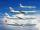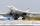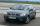The cyclist

The cyclist went from village to town. First half of journey went at 20 km/h. The second half of the journey, which mostly fell, went at 39 km/h. All journey took 88 minutes. Calculate the distance from the village to the town.

Result

d =  38.8 km

Solution:Leave us a comment of example and its solution (i.e. if it is still somewhat unclear...):Be the first to comment!To solve this example are needed these knowledge from mathematics:

Do you have a system of equations and looking for calculator system of linear equations? Do you want to convert length units?

Next similar examples:

1. AircraftsAbove the town hall tower flew the plane with constant speed 592 km/h and 15 minutes later the second plane at speed of 675 km/h. How long and how far from the town hall will be aircrafts caught up?
2. Car and motorcyclistA car and a motorcyclist rode against each other from a distance of 190 km. The car drove 10km/h higher than the motorcyclist and started half an hour later. It met a motorcyclist in an hour and thirty minutes. Determine their speeds.
3. Theorem proveWe want to prove the sentence: If the natural number n is divisible by six, then n is divisible by three. From what assumption we started?
4. StormSo far, a storm has traveled 35 miles in 1/2 hour in direction straight to observer. If it is currently 5:00 p. m. and the storm is 105 miles away from you, at what time will the storm reach you? Explain how you solved the problem.
5. Blackjack TU160Bomber Tupolev TU160 started at 9:00 from the airport and flew at average speed of 1850 km/h toward the airfield B distant 3500 km. After evaluating the danger from the airport B started at 9:45 B towards A fighter MIG 29 at an average speed 2300 km/h. At
6. Two numbersWe have two numbers. Their sum is 140. One-fifth of the first number is equal to half the second number. Determine those unknown numbers.
7. Three workshopsThere are 2743 people working in three workshops. In the second workshop works 140 people more than in the first and in third works 4.2 times more than the second one. How many people work in each workshop?
8. TheatroTheatrical performance was attended by 480 spectators. Women were in the audience 40 more than men and children 60 less than half of adult spectators. How many men, women and children attended a theater performance?
9. Factory and divisionsThe factory consists of three auxiliary divisions total 2,406 employees. The second division has 76 employees less than 1st division and 3rd division has 212 employees more than the 2nd. How many employees has each division?
10. Three unknownsSolve the system of linear equations with three unknowns: A + B + C = 14 B - A - C = 4 2A - B + C = 0
11. Elimination methodSolve system of linear equations by elimination method: 5/2x + 3/5y= 4/15 1/2x + 2/5y= 2/15
12. WavelengthCalculate the wavelength of the tone frequency 11 kHz if the sound travels at speeds of 343 m/s.
13. Linear systemSolve this linear system (two linear equations with two unknowns): x+y =36 19x+22y=720
14. Freight and passenger carThe truck starts at 8 pm at 30 km/h. Passenger car starts at 8 pm at 40 km/h. Passenger arrives in the destination city 1 hour and 45 min earlier. What is the distance between the city of departure and destination city?
15. Equations - simpleSolve system of linear equations: x-2y=6 3x+2y=4
16. TreesAlong the road were planted 250 trees of two types. Cherry for 60 CZK apiece and apple 50 CZK apiece. The entire plantation cost 12,800 CZK. How many was cherries and apples?
17. PoojaPooja and Deepa age is 4:5, 4 years back it was 8:11. What is the age of Pooja now?